Case Based Questions (MCQ)

Chapter 3 Class 12 Matrices
Serial order wise

## Three schools DPS, CVC and KVS decided to organize a fair for collecting money for helping the flood victims. They sold handmade fans, mats and plates from recycled material at a cost of Rs. 25, Rs.100 and Rs. 50 each respectively. The numbers of articles sold are given as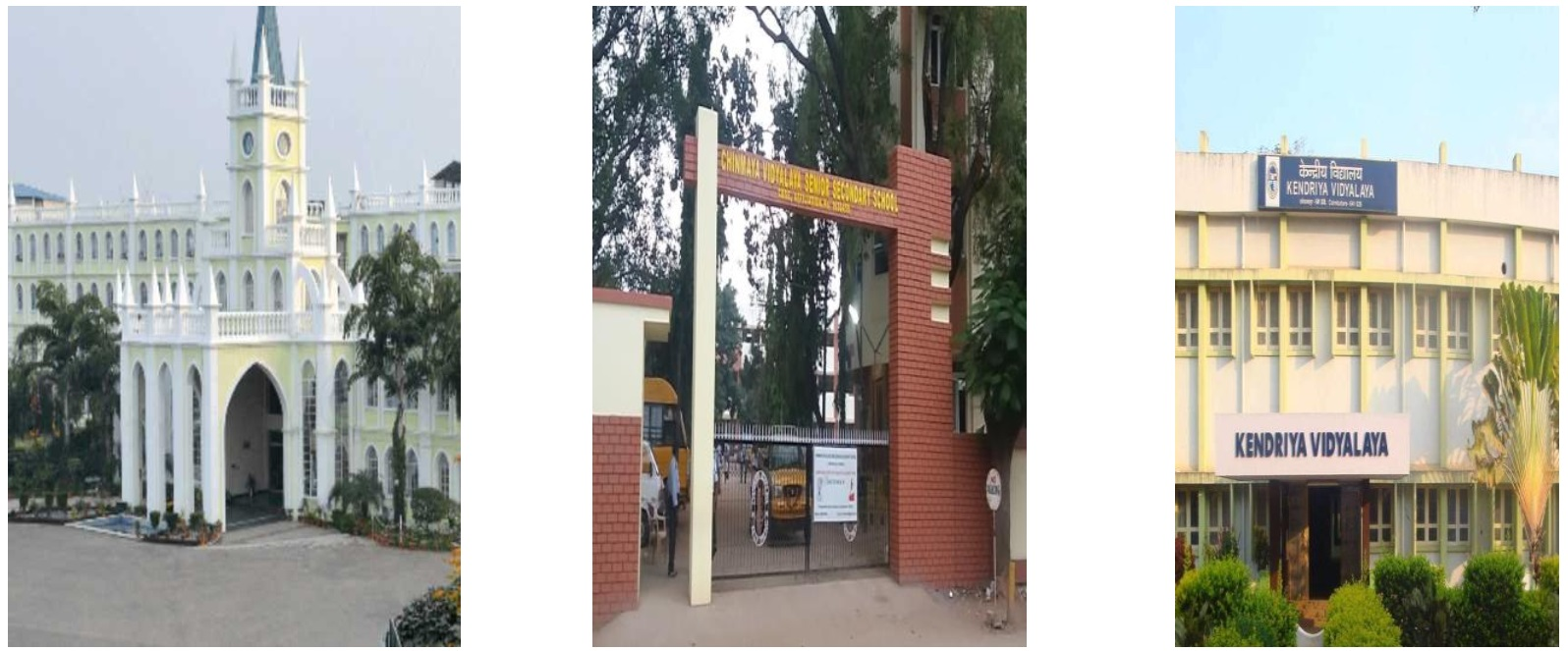School /Article DPS CVC KVS Handmade fans 40 25 35 Mats 50 40 50 Plates 20 30 40

## Based on the information given above, and the following questions: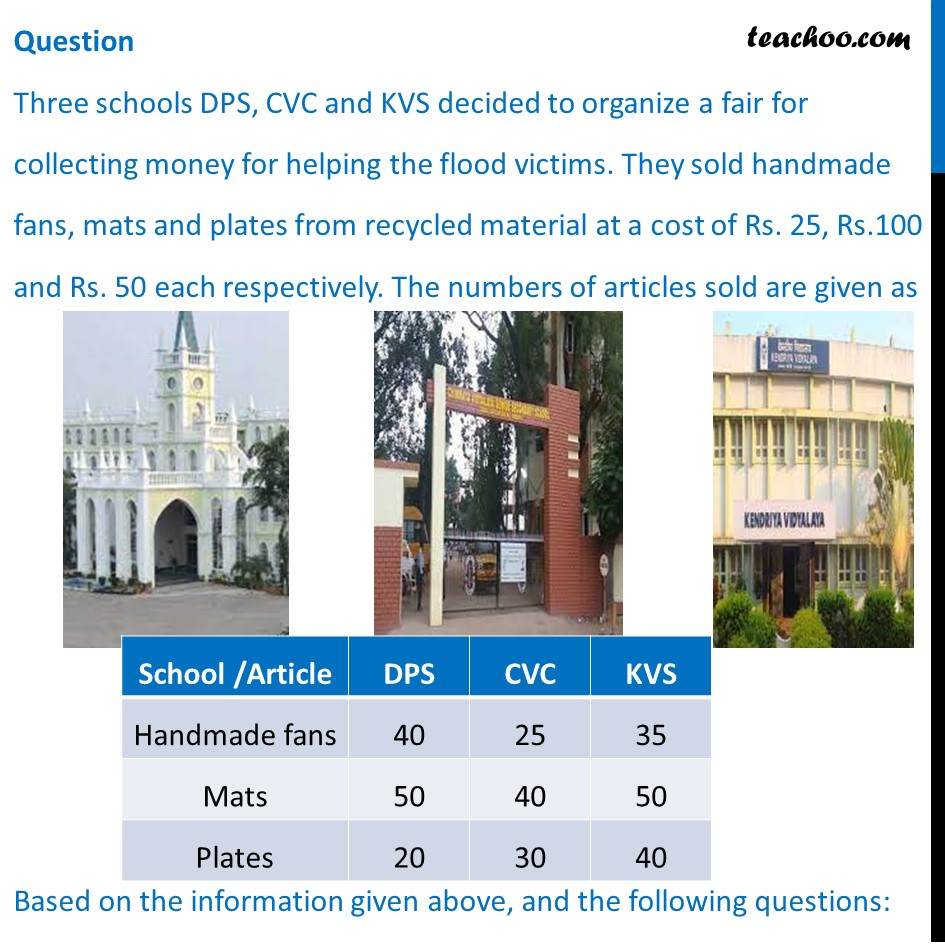## (a) 700 (b) 7,000 (c) 6,125 (d) 7,875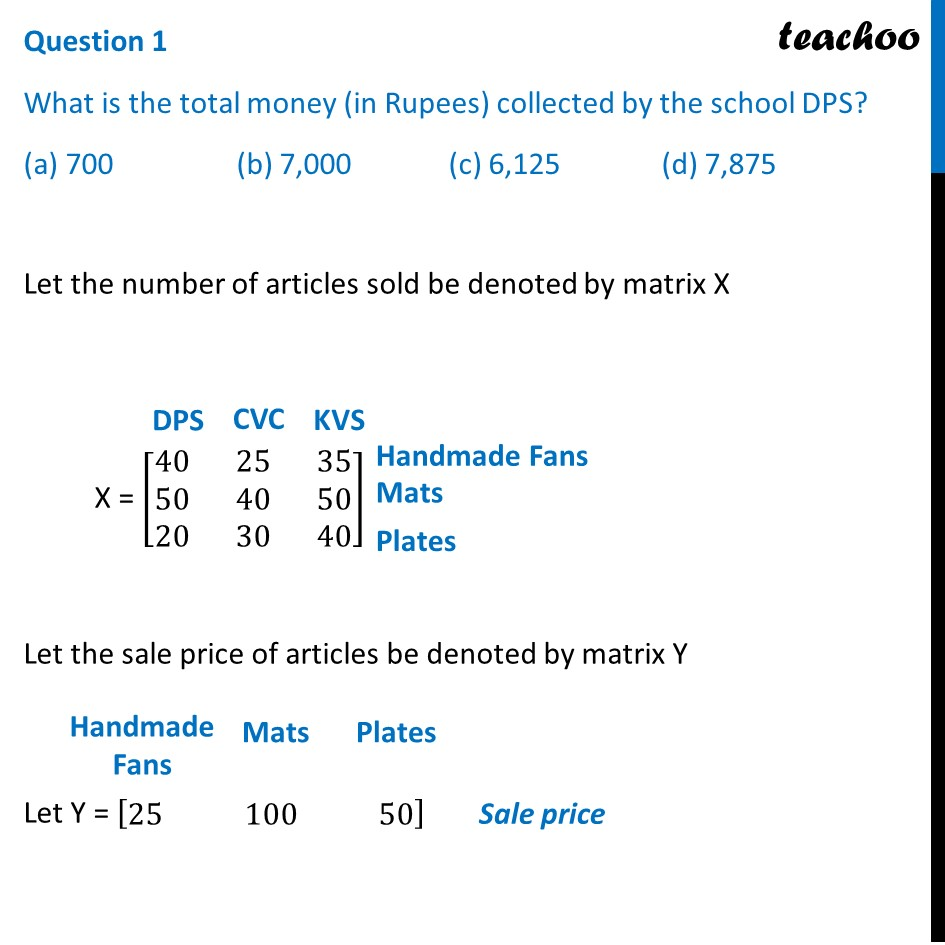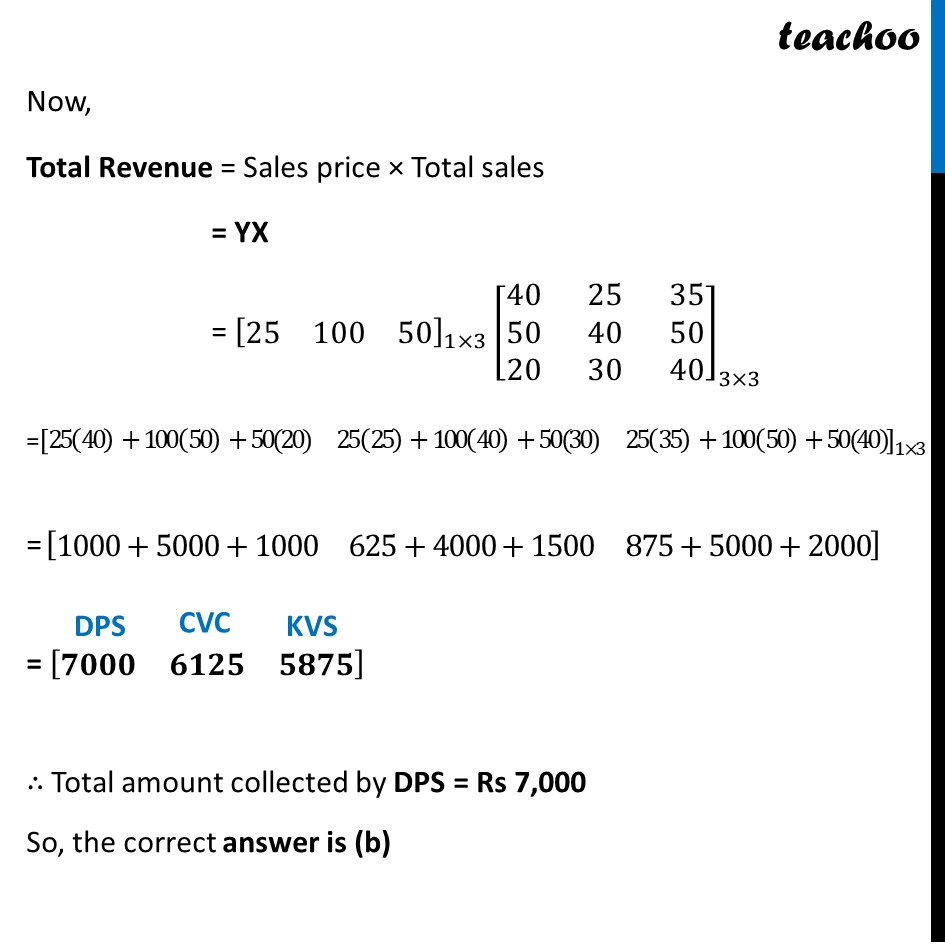## (a) 14,000 (b) 15,725 (c) 21,000 (d) 13,125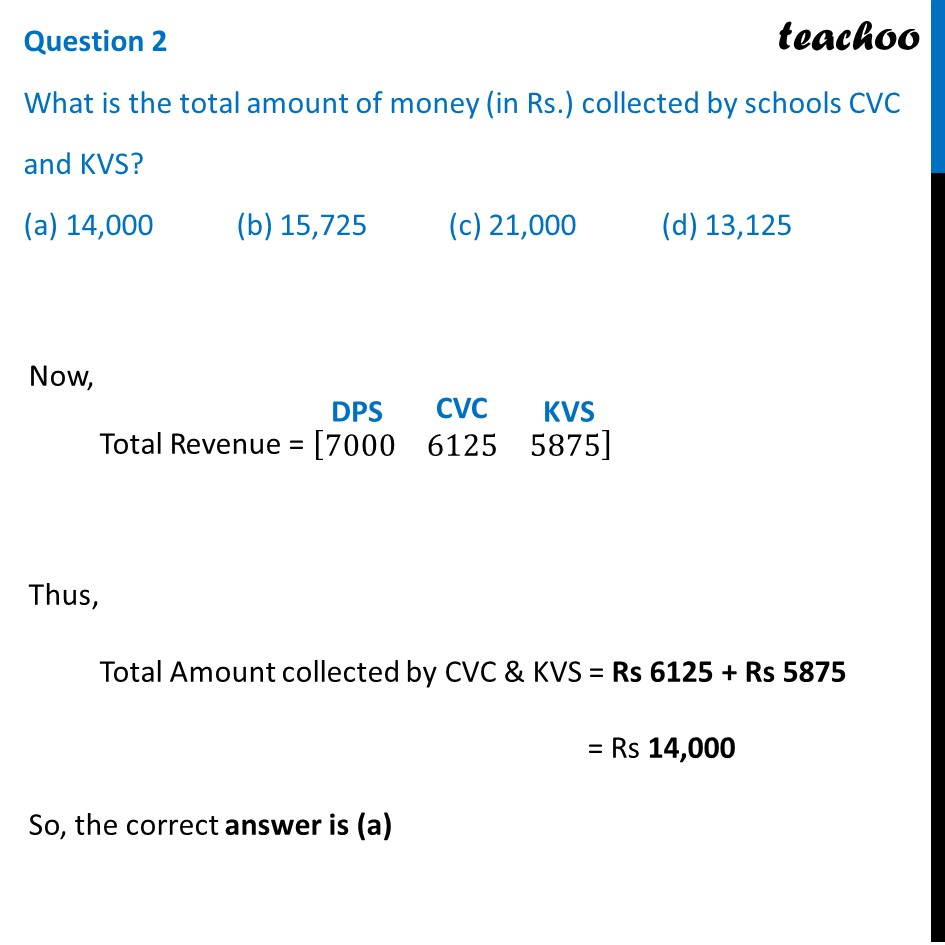## (a) Rs. 15,775 (b) Rs. 14,000 (c) Rs. 21,000 (d) Rs. 17,125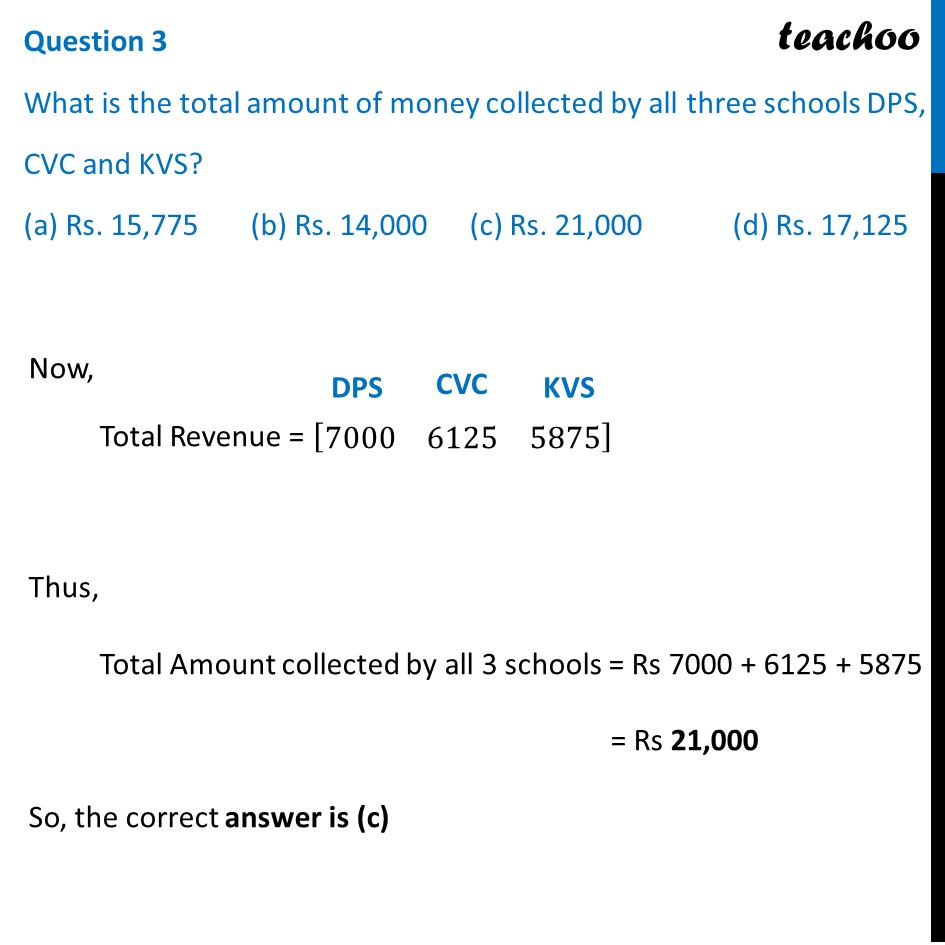## (a) Rs. 18,000 (b) Rs. 6,750 (c) Rs. 5,000 (d) Rs. 21,250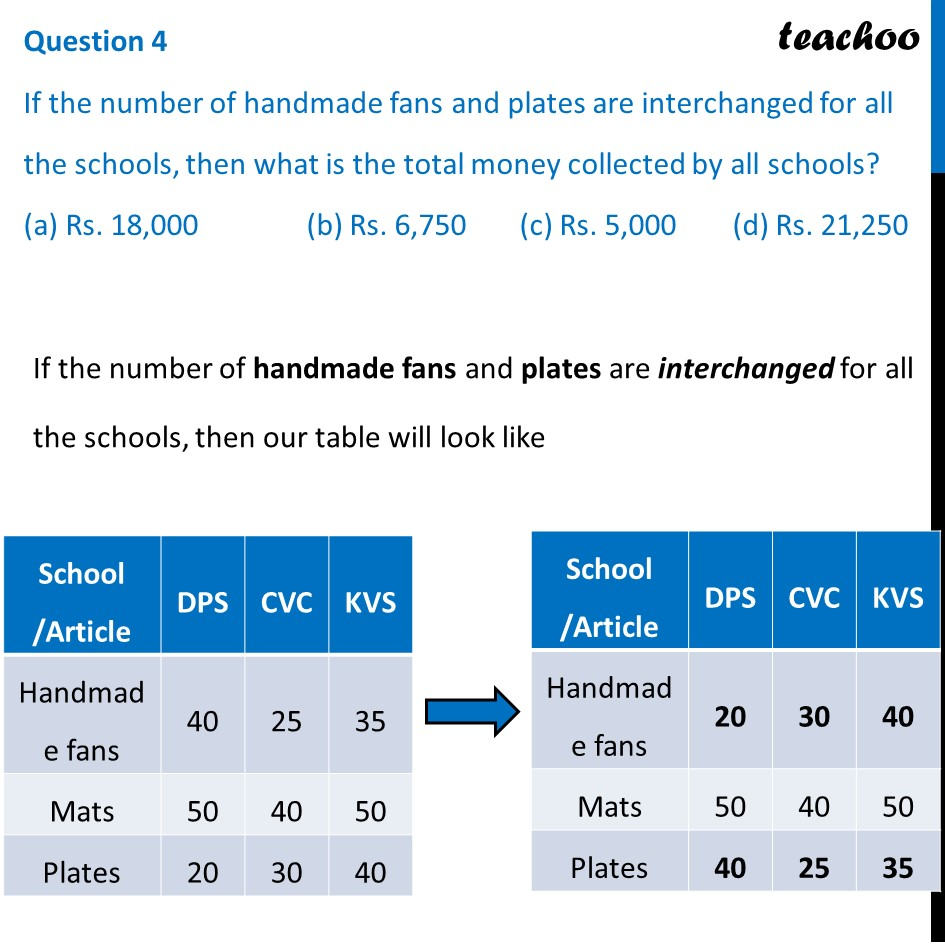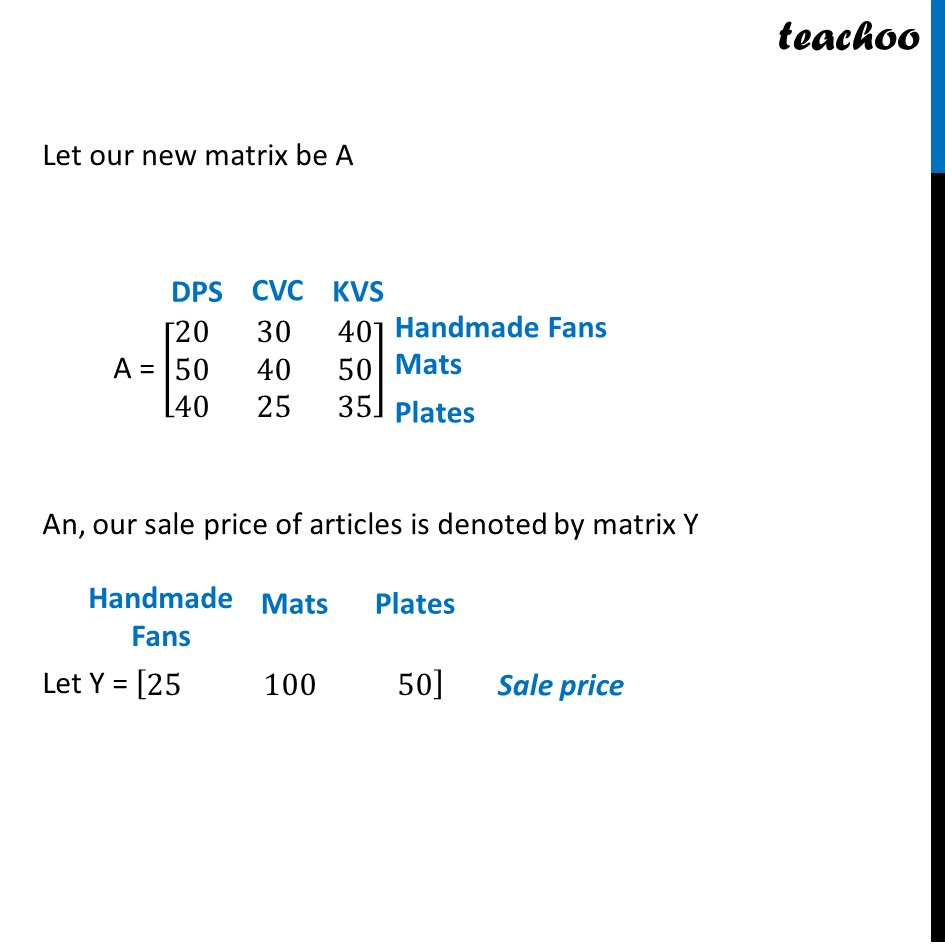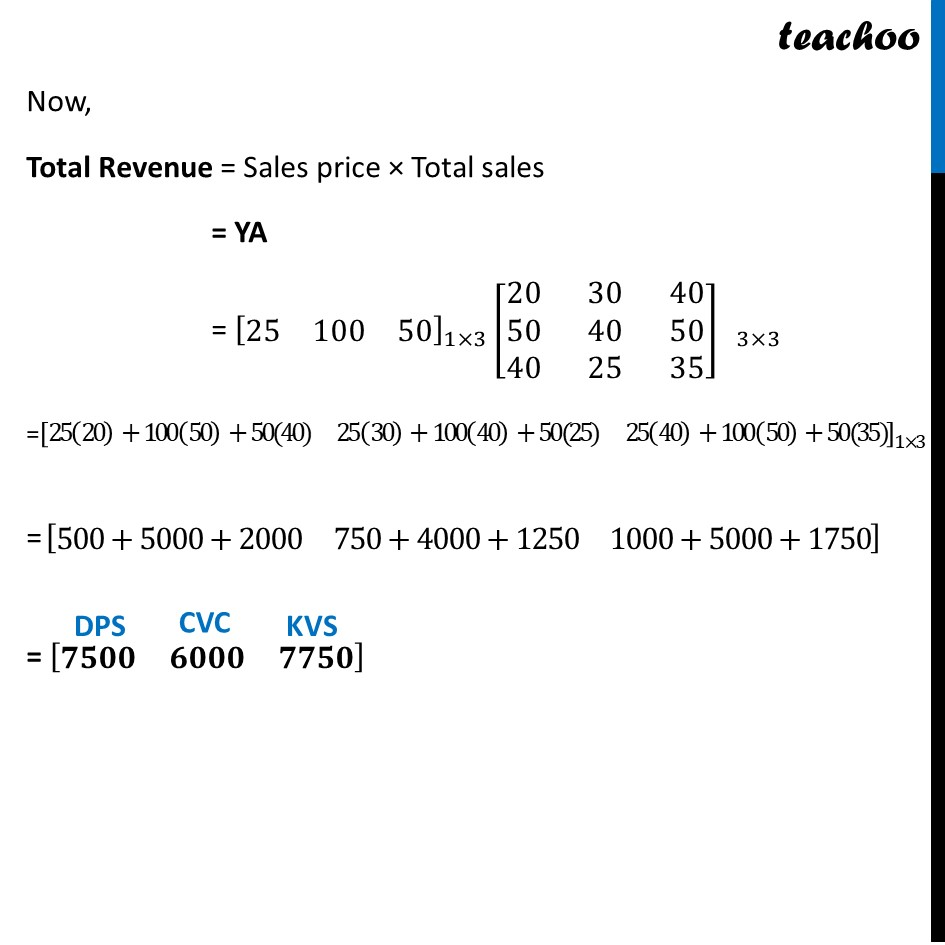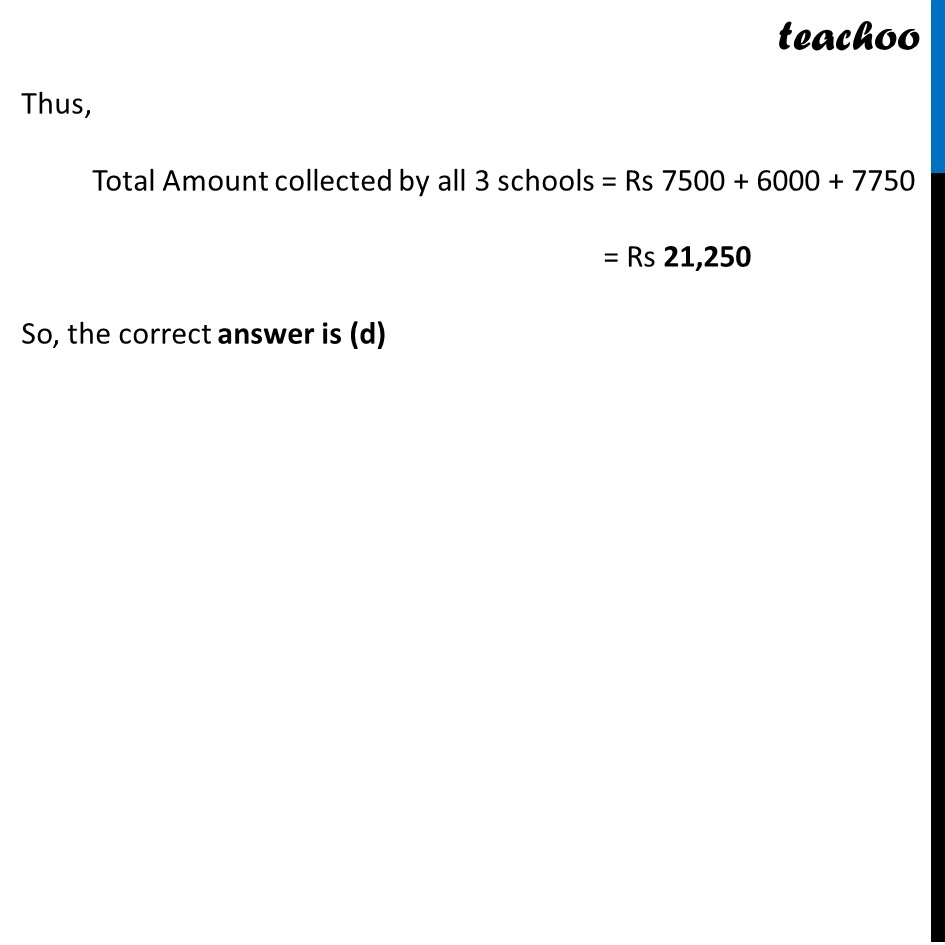## (a) 230 (b) 130 (c) 430 (d) 330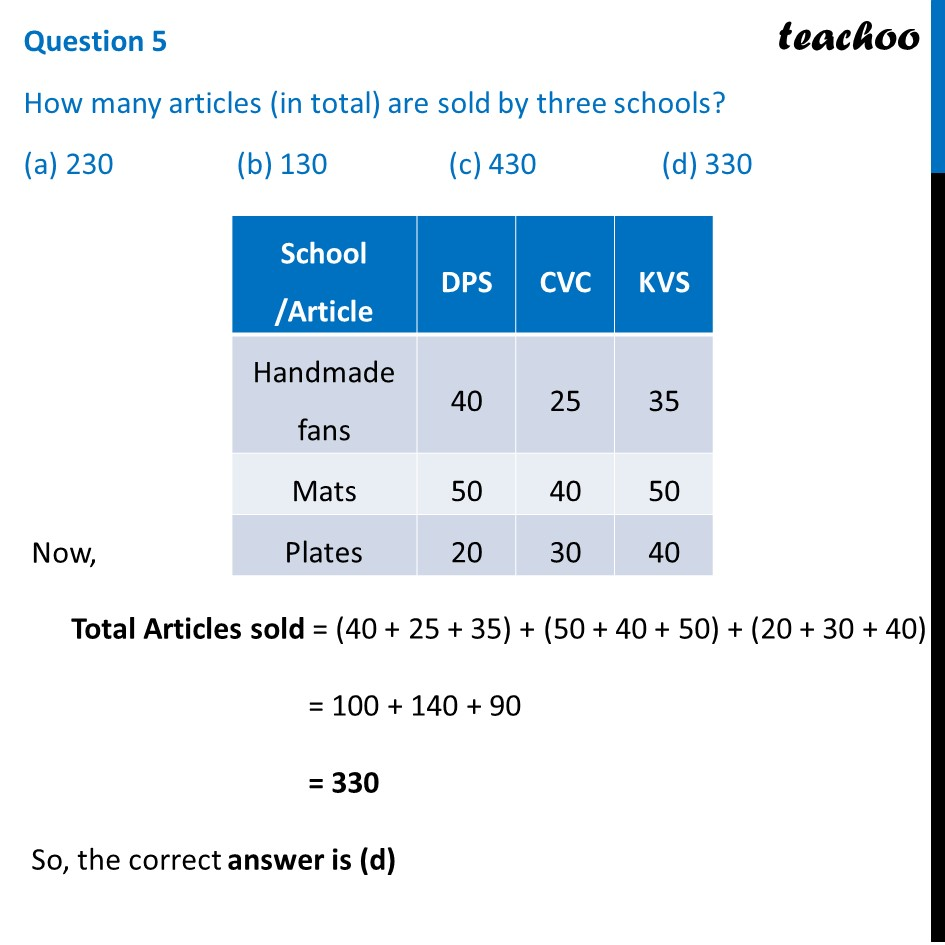Learn in your speed, with individual attention - Teachoo Maths 1-on-1 Class

### Transcript

Question Three schools DPS, CVC and KVS decided to organize a fair for collecting money for helping the flood victims. They sold handmade fans, mats and plates from recycled material at a cost of Rs. 25, Rs.100 and Rs. 50 each respectively. The numbers of articles sold are given as Based on the information given above, and the following questions: Question 1 What is the total money (in Rupees) collected by the school DPS? (a) 700 (b) 7,000 (c) 6,125 (d) 7,875 Let the number of articles sold be denoted by matrix X X = [■8(40& 25& 35@50& 40& 50@20& 30& 40)] Let the sale price of articles be denoted by matrix Y Let Y = [■8(25& 100& 50)] Now, Total Revenue = Sales price × Total sales = YX = [■8(25&100&50)]_(1×3) [■8(40& 25& 35@50& 40& 50@20& 30& 40)]_(3×3) = [■8(25(40)+100(50)+50(20)&25(25)+100(40)+50(30)&25(35)+100(50)+50(40))]_(1×3) = [■8(1000+5000+1000&625+4000+1500&875+5000+2000)] = [■8(𝟕𝟎𝟎𝟎&𝟔𝟏𝟐𝟓&𝟓𝟖𝟕𝟓)] ∴ Total amount collected by DPS = Rs 7,000 So, the correct answer is (b) Question 2 What is the total amount of money (in Rs.) collected by schools CVC and KVS? (a) 14,000 (b) 15,725 (c) 21,000 (d) 13,125 Now, Total Revenue = [■8(7000&6125&5875)] Thus, Total Amount collected by CVC & KVS = Rs 6125 + Rs 5875 = Rs 14,000 So, the correct answer is (a) Question 3 What is the total amount of money collected by all three schools DPS, CVC and KVS? (a) Rs. 15,775 (b) Rs. 14,000 (c) Rs. 21,000 (d) Rs. 17,125 Now, Total Revenue = [■8(7000&6125&5875)] Thus, Total Amount collected by all 3 schools = Rs 7000 + 6125 + 5875 = Rs 21,000 So, the correct answer is (c) Question 4 If the number of handmade fans and plates are interchanged for all the schools, then what is the total money collected by all schools? (a) Rs. 18,000 (b) Rs. 6,750 (c) Rs. 5,000 (d) Rs. 21,250 If the number of handmade fans and plates are interchanged for all the schools, then our table will look like Let our new matrix be A A = [■8(20& 30& 40@50& 40& 50@40& 25& 35)] An, our sale price of articles is denoted by matrix Y Let Y = [■8(25& 100& 50)] Now, Total Revenue = Sales price × Total sales = YA = [■8(25&100&50)]_(1×3) 〖[■8(20& 30& 40@50& 40& 50@40& 25& 35)] " " 〗_(3×3) = [■8(25(20)+100(50)+50(40)&25(30)+100(40)+50(25)&25(40)+100(50)+50(35))]_(1×3) = [■8(500+5000+2000&750+4000+1250&1000+5000+1750)] = [■8(𝟕𝟓𝟎𝟎&𝟔𝟎𝟎𝟎&𝟕𝟕𝟓𝟎)] Thus, Total Amount collected by all 3 schools = Rs 7500 + 6000 + 7750 = Rs 21,250 So, the correct answer is (d) Question 5 How many articles (in total) are sold by three schools? (a) 230 (b) 130 (c) 430 (d) 330 Now, Total Articles sold = (40 + 25 + 35) + (50 + 40 + 50) + (20 + 30 + 40) = 100 + 140 + 90 = 330 So, the correct answer is (d)